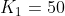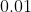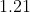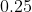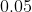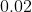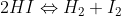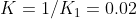# AP Chemistry : Properties of the Equilibrium Constant

## Example Questions

### Example Question #1 : Properties Of The Equilibrium Constant

Calculate the equilibrium constant for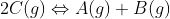given the following information: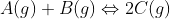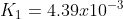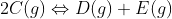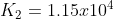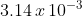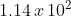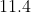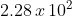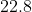Explanation: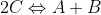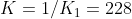### Example Question #51 : Chemical Equilibrium

Calculate the equilibrium constant for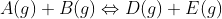given the following information: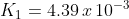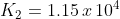73.2

0.143

15.8

50.5

25.3

50.5

Explanation: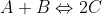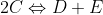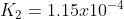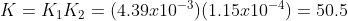### Example Question #51 : Chemical Equilibrium

Calculate the equilibrium constant for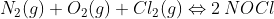given the following information: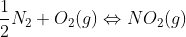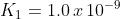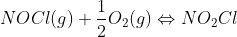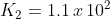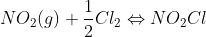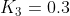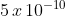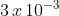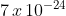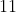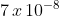Explanation: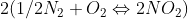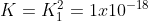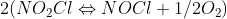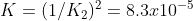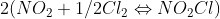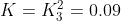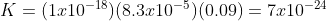### Example Question #1 : Properties Of The Equilibrium Constant

Calculate the equilibrium constant for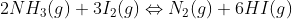given the following information: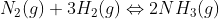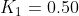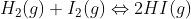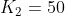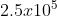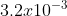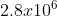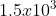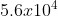Explanation: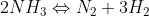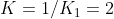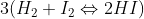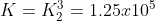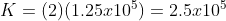### Example Question #2 : Properties Of The Equilibrium Constant

Calculate the equilibrium constant for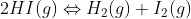given the following information: